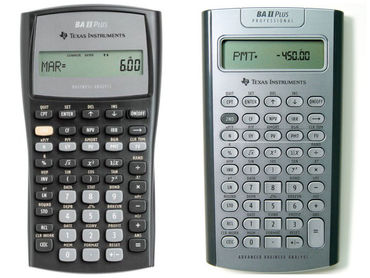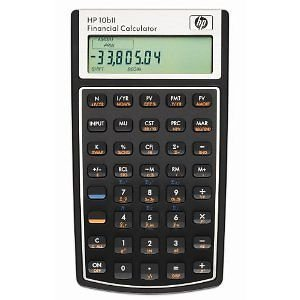Skip to main content
It looks like you're using Internet Explorer 11 or older. This website works best with modern browsers such as the latest versions of Chrome, Firefox, Safari, and Edge. If you continue with this browser, you may see unexpected results.

# Finance: Time Value of Money

## Learn about Time Value of Money

All the financial calculations in finance are based upon compounding rates. In addition, Time Value of Money has applications in many areas of finance including capital Budgeting, bond valuation, and stock valuation. Future Value describes the process of finding what an investment today will grow to in the future. This is called compounding. Present Value describes the process of determining what a cash flow to be received in the future is worth in today's dollars. This is referred to as discounting.  Therefore time value of money calculations finds the value of present value which is equal to the future value at a given interest rate and number of periods of time.

## Apps, Excel, and Calculators

Even though most financial calculations can be accomplished using basic math and elementary algebra it is recommended you use a good calculator, app, or an excel spreadsheet.  Here are a few methods to calculate time value of money problems.  In addition, there will be tutorials to use each.   My recommendation if you don’t already own a calculator is to use either excel or a phone app.

The TI-84 is a graphing calculator; however, it will perform many financial calculations.This is the BA-II calculator made by Texas Instruments for business students.   It is more versatile and will calculate every financial problem.This is Hewlett Packard version of a business calculator.Excel is free to Sullivan University students and a wonderful tool in calculating all time value of money calculations.Here is a website for a great phone app.  This app along with your excel spread sheet will calculate all financial problems for free.

http://www.fncalculator.com/